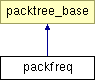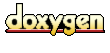Main Page   Class Hierarchy   Compound List   File List   Compound Members   File Members

# packfreq Class Reference

Build a wavelet packet tree for frequency analysis. More...

`#include <packfreq.h>`

Inheritance diagram for packfreq::List of all members.

## Public Methods

packfreq (const double *vec, const size_t n, liftbase< packcontainer, double > *w)
Construct a wavelet packet tree from a vector of double values. More...

~packfreq ()
destructor does nothing. More...

void getLevel (const size_t level)
Build a level basis matrix. More...

void plotMat (const size_t N)
Print out the level basis matrix so that it can be plotted as a three dimensional surface. More...

void prMat ()
Print the contents of the level basis matrix. More...

## Protected Methods

packfreq (const packfreq &rhs)
disallow the copy constructor. More...

packfreq ()
disallow the default constructor. More...

## Private Methods

void findLevel (packnode< double > *top, size_t cur_level, const size_t level)
Traverse the tree, from left to right, and add a node at level to the level basis matrix. More...

## Private Attributes

GrowableArray<packnode<double> *> mat
Level basis matrix. More...

## Detailed Description

Build a wavelet packet tree for frequency analysis.

Wavelet frequency analysis uses a modified wavelet packet tree. Horizontal slices through the modified wavelet packet tree (the so called "level basis") are ordered in increasing frequency regions.

This class and the standard wavelet packet tree class are derived from the same base class. The standard wavelet class includes functions to calculate a minimal data representation relative to a cost function.

The constructor for this class is passed two arguments:

1. A vector of doubles containing the data set (e.g., the signal). The length of the vector must be a power of two.
2. A pointer to a wavelet lifting scheme class that will be used in calculating the wavelet transform step.

If the vector passed to the constructor contains N double values, the result of the constructor will be a wavelet packet tree with log2(N) levels.

Author:
Ian Kaplan

Definition at line 78 of file packfreq.h.

## Constructor & Destructor Documentation

 packfreq::packfreq ( const packfreq & rhs )` [inline, protected]`
 disallow the copy constructor. Definition at line 89 of file packfreq.h.```00089 {}; ```

 packfreq::packfreq ( )` [inline, protected]`
 disallow the default constructor. Definition at line 91 of file packfreq.h.```00091 {}; ```

 packfreq::packfreq ( const double * vec, const size_t n, liftbase< packcontainer, double > * w )
 Construct a wavelet packet tree from a vector of double values. The size of the vector, which must be a power of two, is passed in N. A pointer to a wavelet Lifting Scheme object is passed in w. The wavelet Lifting Scheme object is used to calculate the wavelet transform step which is applied at each level (where level > 0) of the wavelet packet tree. The first level (level 0) of the wavelet packet tree contains the original data set. The "newLevel" function is defined in the base class. This function is used to calculate both the standard and the modified wavelet packet trees. The modified wavelet packet tree is calculated by this class and is used for Wavelet frequency analysis. The wavelet transform step uses two filters H and G to calculate the low pass (scaling function) and the high pass (wavelet function). In the standard transform the low pass result is always placed in the lower half of the array and the high pass result is placed in the upper half of the array. Each of these two half arrays form the input for the next step. In the modified transform calculated by this class, the right child uses the standard algorithm where H and G results are in the upper and lower halves of the result array. However, the left child inverts the locations of the H and G results, placing the result of the G filter in the lower half and the result of the H filter in the upper half. The two boolean flags that are passed to the newLevel function determine whether a frequency ordered wavelet packet tree is calculated and whether the location of the filter results is inverted. vec An array of double values on which the wavelet packet transform is calculated. N The number of elements in the input array w A pointer to the the wavelet transform object to use in calculating the wavelet packet transform. Definition at line 85 of file packfreq.cpp.```00088 { 00089 waveObj = w; 00090 00091 block_pool mem_pool; 00092 double *vecCopy = (double *)mem_pool.pool_alloc( N * sizeof( double ) ); 00093 00094 for (int i = 0; i < N; i++) { 00095 vecCopy[i] = vec[i]; 00096 } 00097 00098 root = new packnode( vecCopy, N, packnode::OriginalData ); 00099 root->mark( true ); 00100 // 00101 // The first level uses the standard wavelet calculation, so 00102 // reverse = false 00103 // freqCalc, reverse 00104 newLevel( root, true, false ); 00105 } // packfreq ```

 packfreq::~packfreq ( )` [inline]`
 destructor does nothing. Definition at line 99 of file packfreq.h.```00099 {} ```

## Member Function Documentation

 void packfreq::findLevel ( packnode< double > * top, size_t cur_level, const size_t level )` [private]`
 Traverse the tree, from left to right, and add a node at level to the level basis matrix. Levels are numbered from 0 at the root. Definition at line 115 of file packfreq.cpp. Referenced by getLevel(). ```00118 { 00119 if (top != 0) { 00120 if (cur_level == level) { 00121 mat.append(top); 00122 } 00123 else { 00124 findLevel( top->lhsChild(), cur_level+1, level ); 00125 findLevel( top->rhsChild(), cur_level+1, level ); 00126 } 00127 } 00128 } // findLevel ```

 void packfreq::getLevel ( const size_t level )
 Build a level basis matrix. The "level basis" is a horizontal slice through the wavelet packet tree. Here the levels are numbered from zero at root (which contains the original data). The most useful basis for frequency analysis is a square matrix. If the original data set consists of 1024 elements, level 5 of the tree consists of 32 tree nodes containing 32 elements each. ``` number of elements in a tree node level number of nodes 1024 0 1 512 1 2 256 2 4 128 3 8 64 4 16 32 5 32 ``` The level basis is built from left to right, in the horizontal slice through the tree. The left most node contains the lowest frequency band. Definition at line 158 of file packfreq.cpp. Referenced by main(). ```00159 { 00160 findLevel( root, 0, level ); 00161 } // getLevel ```

 void packfreq::plotMat ( const size_t N )
 Print out the level basis matrix so that it can be plotted as a three dimensional surface. The level basis for a given level is constructed by the getLevel() function. In the discussion of this function below, we will consider the case where the modified wavelet packet tree was built from a data set of 1024 elements and the level basis was at level 5, resulting in a matrix of 32 nodes, each of which has 32 values. mat is the left most node (and lowest frequency band), mat is the right most (and highest frequency band). The values (*mat) ... (*mat) represent frequency values at particular time intervals. If we use two variables, x and y, to index the matrix then (*mat[y])[x] defines a time/frequency plane, where the x-index is time and the y-index is frequency. The value at (*mat[y])[x] is the magnitude a particular time/frequency point. In plotting a surface from this data, time or frequency are plotted on the x or y axis. The magnitude at (*mat[y])[x] is plotted on the Z-axis. Following Ripples in Mathematics by Jensen and la-Cour Harbo (Springer Verlag, 2001), the calculation for the z-axis value is: ``` m = (*mat)[y][x] z_val = log( 1 + val^2 ) ``` Where log is the natural log. This function has been used to generate data for gnuPlot surface plots. Whether frequency is plotted on the x-axis or the y-axis depends on the nature of the data. If a signal line sin(x) is analyzed, the frequency is constant. To avoid the ridge produced by the sin(x) frequency obscuring the surface, it is better to plot time on the y-axis and frequency on the x-axis. In the case of a constantly changing frequency (like the linear chirp), time is plotted on the x-axis and frequency is plotted on the y-axis. Another wrinkle involves the values plotted on the x and y axis. The simplest plot of time (say on the x-axis) would consist of the 32 time regions (e.g., (*mat[y][x])). Similarly, frequency would consist of 32 frequency regions (on the y-axis). This would yield a surface numbered from 0..31 on both the x and y axis. The axis labels can be scaled to represent the frequency and time ranges. This function was used to generate different plots, so various parts are commented out, making it a bit of a hack. Definition at line 228 of file packfreq.cpp. Referenced by main(). ```00229 { 00230 size_t num_y = mat.length(); 00231 const double incr = (double)N / (double)num_y; 00232 if (num_y > 0) { 00233 size_t num_x = mat->length(); 00234 00235 double freq_start = 0.0; 00236 for (size_t y = 0; y < num_y; y++) { 00237 double time_start = 0.0; 00238 for (size_t x = 0; x < num_x; x++) { 00239 double val = (*mat[y])[ x ]; 00240 // plot time on the x, frequency on y 00241 // printf(" %d %d %7.4f\n", x, y, log(1+(val*val)) ); 00242 00243 // plot frequency on x, time on y 00244 printf(" %d %d %7.4f\n", y, x, log(1+(val*val)) ); 00245 00246 // plot actual frequency and time values, 00247 // with frequency on x and time on y 00248 // printf(" %7.4f %7.4f %7.4f\n", 00249 // freq_start, time_start, log(1+(val*val)) ); 00250 00251 time_start = time_start + incr; 00252 } 00253 freq_start = freq_start + incr; 00254 printf("\n"); 00255 } 00256 } 00257 } // plotMat ```

 void packfreq::prMat ( )
 Print the contents of the level basis matrix. Definition at line 264 of file packfreq.cpp.```00265 { 00266 int num_y = mat.length(); 00267 if (num_y > 0) { 00268 size_t num_x = mat->length(); 00269 for (int y = num_y-1; y >= 0; y--) { 00270 for (size_t x = 0; x < num_x; x++) { 00271 printf(" %7.4f ", (*mat[y])[ x ] ); 00272 } 00273 printf("\n"); 00274 fflush(stdout); 00275 } 00276 } 00277 } // prMat ```

## Member Data Documentation

 GrowableArray< packnode< double > *> packfreq::mat` [private]`
 Level basis matrix. Definition at line 81 of file packfreq.h.

The documentation for this class was generated from the following files:
Generated at Sat Aug 10 13:23:38 2002 for Wavelet Packet Transform and Lossless Compression by1.2.8.1 written by Dimitri van Heesch, © 1997-2001